Search by Topic

Resources tagged with Practical Activity similar to Nine-pin Triangles:

Filter by: Content type:
Age range:
Challenge level:

There are 183 results

Broad Topics > Using, Applying and Reasoning about Mathematics > Practical ActivityTri.'s

Age 7 to 11 Challenge Level:

How many triangles can you make on the 3 by 3 pegboard?Egyptian Rope

Age 7 to 11 Challenge Level:

The ancient Egyptians were said to make right-angled triangles using a rope with twelve equal sections divided by knots. What other triangles could you make if you had a rope like this?Putting Two and Two Together

Age 7 to 11 Challenge Level:

In how many ways can you fit two of these yellow triangles together? Can you predict the number of ways two blue triangles can be fitted together?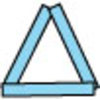Sticks and Triangles

Age 7 to 11 Challenge Level:

Using different numbers of sticks, how many different triangles are you able to make? Can you make any rules about the numbers of sticks that make the most triangles?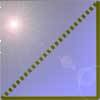Cutting Corners

Age 7 to 11 Challenge Level:

Can you make the most extraordinary, the most amazing, the most unusual patterns/designs from these triangles which are made in a special way?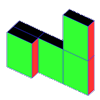Cereal Packets

Age 7 to 11 Challenge Level:

How can you put five cereal packets together to make different shapes if you must put them face-to-face?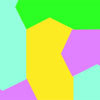Escher Tessellations

Age 7 to 11 Challenge Level:

This practical investigation invites you to make tessellating shapes in a similar way to the artist Escher.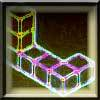Two on Five

Age 5 to 11 Challenge Level:

Take 5 cubes of one colour and 2 of another colour. How many different ways can you join them if the 5 must touch the table and the 2 must not touch the table?Little Boxes

Age 7 to 11 Challenge Level:

How many different cuboids can you make when you use four CDs or DVDs? How about using five, then six?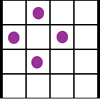Square Corners

Age 7 to 11 Challenge Level:

What is the greatest number of counters you can place on the grid below without four of them lying at the corners of a square?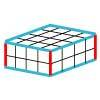Making Cuboids

Age 7 to 11 Challenge Level:

Let's say you can only use two different lengths - 2 units and 4 units. Using just these 2 lengths as the edges how many different cuboids can you make?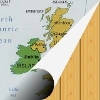Map Folding

Age 7 to 11 Challenge Level:

Take a rectangle of paper and fold it in half, and half again, to make four smaller rectangles. How many different ways can you fold it up?Dice Stairs

Age 7 to 11 Challenge Level:

Can you make dice stairs using the rules stated? How do you know you have all the possible stairs?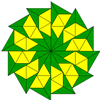Triangle Shapes

Age 5 to 11 Challenge Level:

This practical problem challenges you to create shapes and patterns with two different types of triangle. You could even try overlapping them.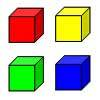Four Colours

Age 5 to 11 Challenge Level:

Kate has eight multilink cubes. She has two red ones, two yellow, two green and two blue. She wants to fit them together to make a cube so that each colour shows on each face just once.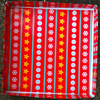Cover the Tray

Age 7 to 11 Challenge Level:

These practical challenges are all about making a 'tray' and covering it with paper.Seven Flipped

Age 7 to 11 Challenge Level:

Investigate the smallest number of moves it takes to turn these mats upside-down if you can only turn exactly three at a time.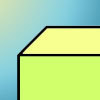Cuboid-in-a-box

Age 7 to 11 Challenge Level:

What is the smallest cuboid that you can put in this box so that you cannot fit another that's the same into it?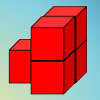Age 7 to 11 Challenge Level:

How can you arrange the 5 cubes so that you need the smallest number of Brush Loads of paint to cover them? Try with other numbers of cubes as well.Creating Cubes

Age 7 to 11 Challenge Level:

Arrange 9 red cubes, 9 blue cubes and 9 yellow cubes into a large 3 by 3 cube. No row or column of cubes must contain two cubes of the same colour.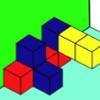Three Sets of Cubes, Two Surfaces

Age 7 to 11 Challenge Level:

How many models can you find which obey these rules?Order the Changes

Age 7 to 11 Challenge Level:

Can you order pictures of the development of a frog from frogspawn and of a bean seed growing into a plant?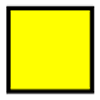Shaping Up

Age 7 to 11 Challenge Level:

Are all the possible combinations of two shapes included in this set of 27 cards? How do you know?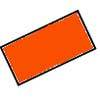Two by One

Age 7 to 11 Challenge Level:

An activity making various patterns with 2 x 1 rectangular tiles.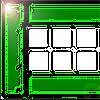Fencing

Age 7 to 11 Challenge Level:

Arrange your fences to make the largest rectangular space you can. Try with four fences, then five, then six etc.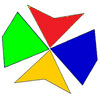Making Maths: Kites and Darts

Age 7 to 11 Challenge Level:

Using these kite and dart templates, you could try to recreate part of Penrose's famous tessellation or design one yourself.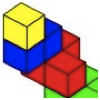Four Layers

Age 5 to 11 Challenge Level:

Can you create more models that follow these rules?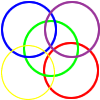Hoops/rope

Age 7 to 11 Challenge Level:

Ideas for practical ways of representing data such as Venn and Carroll diagrams.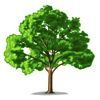How Tall?

Age 5 to 11 Challenge Level:

A group of children are discussing the height of a tall tree. How would you go about finding out its height?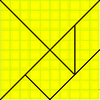Making Maths: Making a Tangram

Age 7 to 11 Challenge Level:

Follow these instructions to make a three-piece and/or seven-piece tangram.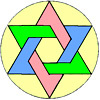Making Maths: Stars

Age 7 to 11 Challenge Level:

Have a go at drawing these stars which use six points drawn around a circle. Perhaps you can create your own designs?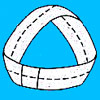Making Maths: Make a Magic Circle

Age 7 to 11 Challenge Level:

Make a mobius band and investigate its properties.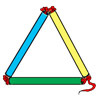Making Maths: Test the Strength of a Triangle

Age 7 to 11 Challenge Level:

Have you noticed that triangles are used in manmade structures? Perhaps there is a good reason for this? 'Test a Triangle' and see how rigid triangles are.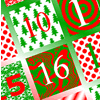Age 5 to 11 Challenge Level:

Our 2008 Advent Calendar has a 'Making Maths' activity for every day in the run-up to Christmas.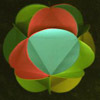Making Maths: Triangular Ball

Age 7 to 11 Challenge Level:

Make a ball from triangles!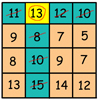Making Maths: Be a Mathemagician

Age 7 to 11 Challenge Level:

Surprise your friends with this magic square trick.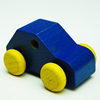Car Journey

Age 7 to 11 Challenge Level:

This practical activity involves measuring length/distance.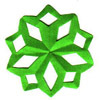Making Maths: Five-point Snowflake

Age 7 to 11 Challenge Level:

Follow these instructions to make a five-pointed snowflake from a square of paper.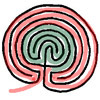Making Maths: A-maze-ing

Age 7 to 11 Challenge Level:

Did you know mazes tell stories? Find out more about mazes and make one of your own.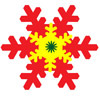Making Maths: Snowflakes

Age 7 to 11 Challenge Level:

It's hard to make a snowflake with six perfect lines of symmetry, but it's fun to try!Cut and Make

Age 7 to 11 Challenge Level:

Cut a square of paper into three pieces as shown. Now,can you use the 3 pieces to make a large triangle, a parallelogram and the square again?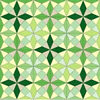A Patchwork Piece

Age 7 to 11 Challenge Level:

Follow the diagrams to make this patchwork piece, based on an octagon in a square.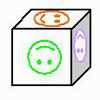Age 7 to 11 Challenge Level:

Make a cube with three strips of paper. Colour three faces or use the numbers 1 to 6 to make a die.Folding

Age 7 to 11 Challenge Level:

What shapes can you make by folding an A4 piece of paper?This Fencing

Age 7 to 11 Challenge Level:

Arrange your fences to make the largest rectangular space you can. Try with four fences, then five, then six etc.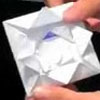Bryony's Triangle

Age 7 to 11 Challenge Level:

Watch the video to see how to fold a square of paper to create a flower. What fraction of the piece of paper is the small triangle?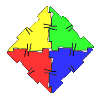Polydron

Age 7 to 11 Challenge Level:

This activity investigates how you might make squares and pentominoes from Polydron.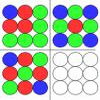Counter Ideas

Age 7 to 11 Challenge Level:

Here are some ideas to try in the classroom for using counters to investigate number patterns.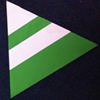Regular Rings 1

Age 7 to 11 Challenge Level:

Can you work out what shape is made by folding in this way? Why not create some patterns using this shape but in different sizes?Square Tangram

Age 7 to 11 Challenge Level:

This was a problem for our birthday website. Can you use four of these pieces to form a square? How about making a square with all five pieces?